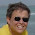## Sunday, June 23, 2013

### Function to collapse some subtrees into a star - while retaining the same total height of the tips

The following was recently requested via the R-sig-phylo mail list serve:

I'd like to collapse the descendants of a node, identified using something like node <- mrca(tree)["A", "B"]. I did not see a function in ape, geiger, phyloch, or picante to do something like collapse.descendants(node). Is there a package with a function like this?

This can be pretty easily done using the functions of phytools. Here's a little function to do this:

function(tree,node){
tt<-splitTree(tree,split=list(node=node,bp=
tree\$edge.length[which(tree\$edge[,2]==node)]))
ss<-starTree(species=tt[]\$tip.label,branch.lengths=
diag(vcv(tt[])))
ss\$root.edge<-0
tree<-paste.tree(tt[],ss)
return(tree)
}

And here is a quick demo:

> tree<-pbtree(n=50,scale=1)
> plotTree(tree,node.numbers=T,fsize=0.8)
> tree<-collapse.to.star(tree,fastMRCA(tree,"t7","t12"))
> tree<-collapse.to.star(tree,fastMRCA(tree,"t50","t18"))
> plotTree(tree,node.numbers=T,fsize=0.8)

Cool. That did exactly what we wanted tit to do. Note that every time we collapse a subtree, the node numbers of the tree will change - so we cannot use the node numbers from the original tree to collapse multiple subtrees (we need to recompute the target node each time).

#### 1 comment:

1.HI,
What about collapsing branches with less than X bootstrap value, so that we do not need to identify every single node in that condition. Can it be done?
Thanks,
Rita

Note: due to the very large amount of spam, all comments are now automatically submitted for moderation.